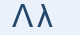Greek Letter Lamda (Λάμδα - Λ)The letter "λ" came from the Semitic alphabet, where it always had the shape of an angle, but its orientation varied. Sometimes the "L"'s would even indicate whether the line was to be read right-to-left or left-to-right. The Greeks used the letter with the angle pointing up. It was turned on its side by the Romans to become the Latin "L".

"Λ" Letter in mathematics and in science

Λ represents:

• the set of logical axioms in the axiomatic method of logical deduction in first-order logic
• the cosmological constant
• a type of baryon

λ represents:

• a unit of measure of volume equal to one micro litre (1 μL) or one cubic milli metre (1 mm3).
• function expressions in the lambda calculus.
• a general eigenvalue in linear algebra.
• the arrival rate in queueing theory
• the average life time or rate parameter in an exponential distribution (commonly used across statistics, physics, and engineering)
• the failure rate in reliability engineering
• the expected number of occurrences in a Poisson distribution in probability
• the lagrange multiplier in the mathematical optimization method, known as the shadow price in economics
• longitude in geodesy
• linear density

Greek words with "λ"

λουλούδι, λόφος